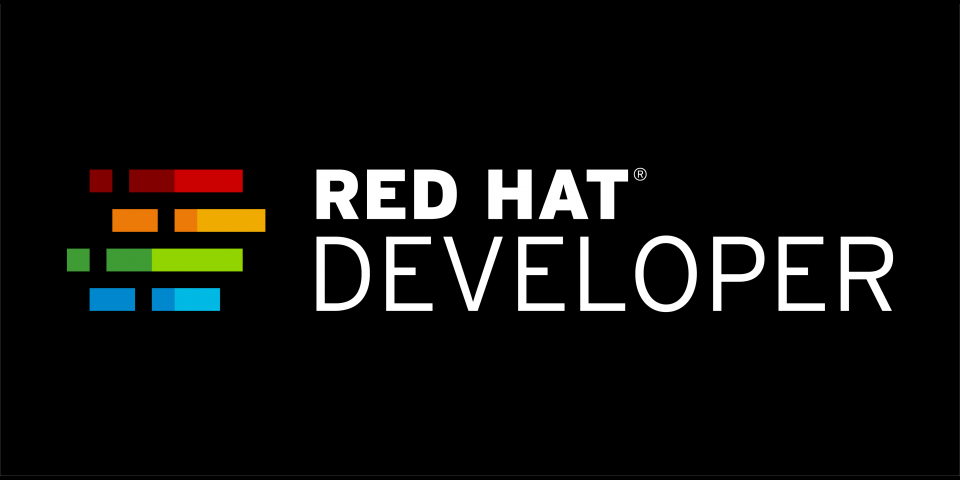You've probably been taught that picking an algorithm that has the best Big-O asymptotic cost will yield the best performance. You might be surprised to find that on current hardware, this isn't always the case. Much of algorithmic analysis assumes very simple costs where the order of operations doesn't matter. Memory access times are assumed to be the same. However, the difference between a cache hit (a few processor clock cycles) and a cache miss that requires access to main memory (a couple hundred cycles) is immense.

This article series is the result of the authors (William Cohen and Ben Woodard) discussion that there is a disconnect on the typical ideas of algorithm efficiency taught in computer science and computer engineering versus what is currently encountered in actual computer systems.

## Introduction

What is the best way to implement a sort? If you paid attention in your algorithms and data structures class you might quickly answer “Quicksort”. However, the correct answer might be more complicated and depend on specific details. No one would ever advocate using the Quicksort algorithm to sort an unordered list containing only two items. This could be more efficiently and simply done with a compare and conditional swap to reorder the elements if needed. The advantages of the Quicksort algorithm would only become apparent when the number of elements to sort is much larger. However, other sorting algorithms such as merge sort might be preferred over the Quicksort algorithm even though both have the same average complexity, O(n log n), due to merge sort having better real-world performance. Incorrect assumptions developers might make during the selection of algorithms and implementing them in programs that can adversely affect performance.

Big-O notation has often been used as the measure of goodness for an algorithm and as a tool to predict how a part of the program will behave in the physical world where programs actually operate. However, the simple sequential models used for big-O analysis do not accurately represent current computer system costs. Modern computer hardware can overlap operations and individual memory access costs can vary immensely (by a factor of one hundred or more) depending on the memory access patterns. High-performance machines can perform multi-element, vector-style operations with much higher throughput than doing the equivalent individual operations separately. The differences between the abstract model and the physical hardware could lead to very noticeable differences in delivered performance. For example iterating through elements in a linked list and array are both O(n) complexity, but on modern processors hardware it is much slower iterating through the elements in a linked list because of the random memory access pattern than iterating through an equivalent one-dimensional array of elements with the regular, easily predicted memory access pattern.

Big-O asymptotic algorithm analysis is showing how the computational work grows in proportional to the problem size growth. However, this does not indicate which algorithm is most efficient for a particular problem size. The lack of constants makes that comparison between algorithms working on a particular problem size difficult. An algorithm that has worse big-O asymptotic performance than another algorithm might have better performance for smaller problem sizes. Given that cache misses cost hundreds of cycles the range of problems sizes where the “worse” algorithm is faster may be surprisingly large. Algorithms such as Coppersmith-Winograd matrix multiplication algorithm (O(n^2.375477)) are theoretically more efficient than the naive implementation of matrix multiply (O(n^3)) but the problem size needs to be so large to provide an advantage that they are not used in practice.

Those constants that are so often ignored for big-O analysis have significant impact on efficiency and power use. For a battery powered tablet playing a video they could make the difference between being able to keep yourself entertained with videos for an entire eight hour transatlantic flight and only being able watch an single two hour movie before the battery is dead. At the other extreme for a high-performance computer system using millions of dollars of electricity each year the power might be a significant portion of the operating cost and there is great pressure to minimize those costs.

Many programmers attempt to use parallel processing to obtain faster performance. At best parallel computers will speed up the program proportional to the number of processors in the system. For parallel computer systems it makes little sense to run a program across multiple processors if it yields no performance improvement over running on a single processor. If care is not taken writing the code, it is quite possible that the overhead to make the program run across multiple processors ends up making the parallel version of the program slower than the basic serial version. You can use your favorite search engine and find many examples of "parallel program slower than sequential program".

Programmers are much more familiar with the simple programming model similar to the ones used for big-O analysis where the order of operations is not important and the operations have the same low costs. For high-performance computers these assumptions do not hold. How operations are grouped can impact cost and there can be orders of magnitudes difference in the cost of operations.

## Coming articles

We're starting a series of articles that will talk about some of the high performance computing issues such as data layout, task granularity, load balancing, and contention. The goal of these articles is to help eliminate some of the assumptions that might have based on traditional simplified program models and steer people toward writing more efficient code for modern computers.

While these topics are typically considered to be in the domain of high performance computing (HPC), this discussion are generally applicable. Each year, features from HPC trickle down to more traditional environments. For example vector processing was only found on supercomputers in the 80's and 90's, but now every virtually every laptop, desktop, and server computer has vector processing support and there are software libraries to use these capabilities.

Last updated: March 26, 2019